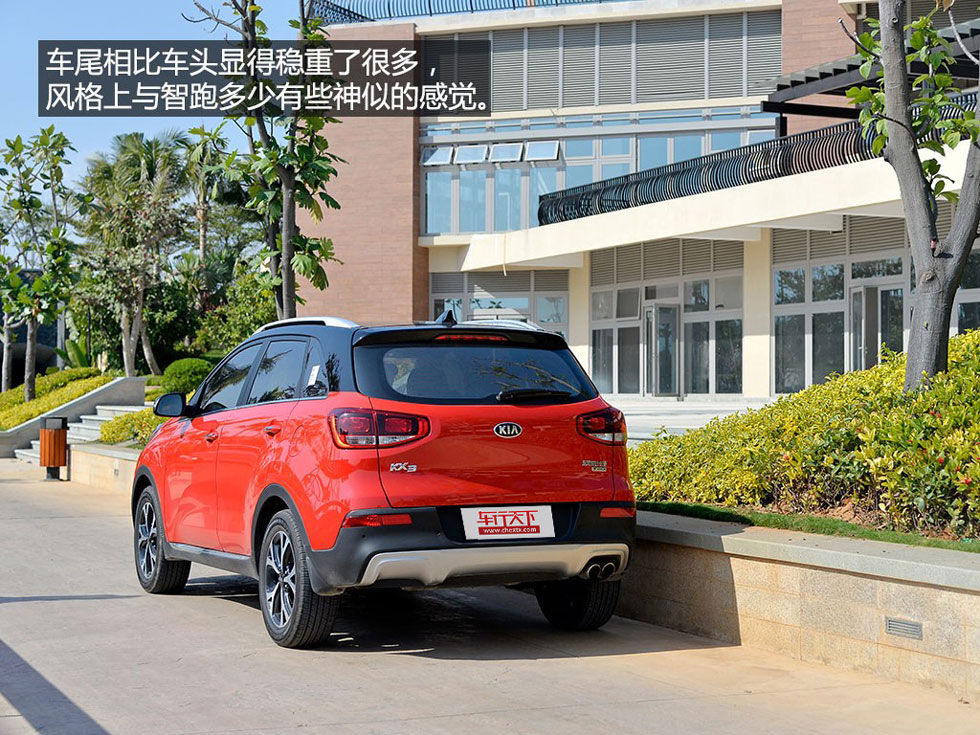# 东风悦达起亚 起亚KX3

## 不可小看 起亚首款小型SUV“傲跑”评测

2015年01月31日 15:27 来源：www.chextx.com 超过：19342次关注 阅读全文

傲跑来了！随着之前KX3概念车的量产，它也有了正式的名字——傲跑，面对已经如此激烈的市场，它来得是否有些晚呢？似乎韩系车慢了半拍，难道是为了战略求稳！北京现代ix25的推出并且表现不俗，起亚才慢步跟上，二者默契有余的同时小型SUV的竞争又多了一位生力军！2014年可以说是小型SUV爆发的一年，以别克昂科拉、标致2008为首的车型开拓了小型SUV这一细分市场。随后，合资、自主品牌纷纷推出自己的产品，让市场的热度急速升温。

从战略意义上，现代与起亚的定位一个是相对中庸，一个是年轻前卫，傲跑就是秉承了起亚的基因由概念车量产而来，外形是相当的夺人眼球！相信大家从之前的相关报道中已经知道了它，从图片上看我多少觉得它的车头样式设计有些过于个性，感觉能接受这样造型的人并不会占大多数，但当我看到实车之后，感觉要好了很多，饱满的车头还是挺招人喜欢的。

虎啸式中网是自彼得希瑞尔加入后带给起亚最好的礼物，现已成为起亚家族最显著的元素之一，作为拥有较强个性的傲跑来说怎能让这样的设计缺席。车身下部从前到后都采用了黑色塑料材质，配合前部的银色护板，样式上给车身增添了几分硬派SUV的味道，也避免了日常的小刮小蹭伤到车漆。

新式的集成大灯承担了更多的指示功能，整体造型比较犀利而精湛，此次试驾的这辆傲跑只是配备了卤素的前大灯，高配车型采用了远近光一体的氙气大灯并配有转向辅助灯。傲跑的LED日间行车灯还兼顾了示宽灯的作用，当大灯开启之后亮度会变弱。

傲跑侧面流线感稍弱，倒是黑雾化的ABC柱让整体车身感觉提升了一个档次，属于同级别的主流水平，车身侧面线条硬朗，前低后高的设计在视觉上营造出较为动感的效果。相比车身线条，双色车身倒是更加吸引人。

傲跑配备的轮胎是耐克森CP672系列的产品，该系列产品还应用于朗动和起亚K3上，这样的搭配还是表现出了傲跑定位于城市的特点。高配车型的备胎规格与其它轮胎规格相同，并且也是铝合金轮圈，低配车型则使用的是非全尺寸备胎。车尾相比车头更显简洁和紧凑，敞开平直的后备箱分界线比较容易装载物品，没有过多的线条修饰，反而更惹人喜欢，显得车尾比较稳重的造型。单边共两出的排气管配合面积更大的银色护板，再加上顶部的鲨鱼鳍天线，让车辆既彰显硬派SUV的气质，还保持着一定的年轻、运动的感觉。

尾灯使用了普通卤素光源，高配车型则采用LED光源。两个方形的设计在夜晚也还是具有着一定的独特性。

还记得起亚的SOUL秀尔吗，拥有较多的流行元素，号称韩国的小mini！此次傲跑车尾在个性化的设计上或许没有车头表现的更加鲜明，但整车双色车身的搭配也让它表现出了时尚、年轻的定位。除了红黑双色搭配外，厂家还提供了蓝白的配色方案，在傲跑的车系当中，只有1.6L、2.0L的顶配车型以及1.6T车型可以选装。

1 2 3 4

#### 相关文章

0-500 字已有评论 0条 查看评论>>

### 起亚KX3### 猜你喜欢

﻿
• 快速找车
• 选择品牌
• 选择品牌
• A  奥迪
• A  阿斯顿·马丁
• A  阿尔法·罗密欧
• B  宝沃
• B  布加迪
• B  巴博斯
• B  保时捷
• B  宾利
• B  奔驰
• B  宝马
• B  本田
• B  别克
• B  标致
• B  比亚迪
• B  宝骏
• B  北汽制造
• B  北汽新能源
• B  北汽幻速
• B  北汽威旺
• B  北京汽车
• B  奔腾
• B  北汽绅宝
• C  长安
• C  长安商用
• C  长城
• C  昌河
• D  大众
• D  道奇
• D  DS
• D  东南
• D  东风风神
• D  东风风行
• D  东风小康
• D  东风风度
• D  东风
• F  福特
• F  丰田
• F  菲亚特
• F  法拉利
• F  福田
• F  福迪
• F  福汽启腾
• G  观致
• G  广汽传祺
• G  广汽吉奥
• G  GMC
• H  红旗
• H  汉腾汽车
• H  哈弗
• H  哈飞
• H  海格
• H  海马
• H  华颂
• H  黄海
• H  华泰
• H  恒天
• J  吉利汽车
• J  捷豹
• J  Jeep
• J  江淮
• J  江铃
• J  金杯
• J  九龙
• J  金旅
• K  凯翼
• K  凯迪拉克
• K  克莱斯勒
• K  科尼塞克
• K  卡威
• K  开瑞
• L  路虎
• L  林肯
• L  劳斯莱斯
• L  兰博基尼
• L  雷克萨斯
• L  铃木
• L  雷诺
• L  理念
• L  力帆
• L  莲花汽车
• L  猎豹
• L  路特斯
• L  陆风
• M  马自达
• M  MG
• M  MINI
• M  玛莎拉蒂
• M  摩根
• M  迈凯轮
• N  纳智捷
• O  欧宝
• O  讴歌
• O  欧朗
• Q  奇瑞
• Q  起亚
• Q  启辰
• R  日产
• R  荣威
• R  瑞麒
• S  三菱
• S  斯威汽车
• S  萨博
• S  smart
• S  斯柯达
• S  斯巴鲁
• S  思铭
• S  双龙
• S  上汽大通
• S  双环
• T  特斯拉
• T  腾势
• W  沃尔沃
• W  五菱汽车
• W  五十铃
• W  威兹曼
• W  威麟
• X  现代
• X  雪佛兰
• X  雪铁龙
• X  西雅特
• Y  一汽
• Y  英菲尼迪
• Y  英致
• Y  依维柯
• Y  野马汽车
• Y  永源
• Z  众泰
• Z  中华
• Z  中兴
• Z  知豆
• 选择车系
• 选择车系
• 车型对比
• 选择品牌
• 选择品牌
• A  奥迪
• A  阿斯顿·马丁
• A  阿尔法·罗密欧
• B  宝沃
• B  布加迪
• B  巴博斯
• B  保时捷
• B  宾利
• B  奔驰
• B  宝马
• B  本田
• B  别克
• B  标致
• B  比亚迪
• B  宝骏
• B  北汽制造
• B  北汽新能源
• B  北汽幻速
• B  北汽威旺
• B  北京汽车
• B  奔腾
• B  北汽绅宝
• C  长安
• C  长安商用
• C  长城
• C  昌河
• D  大众
• D  道奇
• D  DS
• D  东南
• D  东风风神
• D  东风风行
• D  东风小康
• D  东风风度
• D  东风
• F  福特
• F  丰田
• F  菲亚特
• F  法拉利
• F  福田
• F  福迪
• F  福汽启腾
• G  观致
• G  广汽传祺
• G  广汽吉奥
• G  GMC
• H  红旗
• H  汉腾汽车
• H  哈弗
• H  哈飞
• H  海格
• H  海马
• H  华颂
• H  黄海
• H  华泰
• H  恒天
• J  吉利汽车
• J  捷豹
• J  Jeep
• J  江淮
• J  江铃
• J  金杯
• J  九龙
• J  金旅
• K  凯翼
• K  凯迪拉克
• K  克莱斯勒
• K  科尼塞克
• K  卡威
• K  开瑞
• L  路虎
• L  林肯
• L  劳斯莱斯
• L  兰博基尼
• L  雷克萨斯
• L  铃木
• L  雷诺
• L  理念
• L  力帆
• L  莲花汽车
• L  猎豹
• L  路特斯
• L  陆风
• M  马自达
• M  MG
• M  MINI
• M  玛莎拉蒂
• M  摩根
• M  迈凯轮
• N  纳智捷
• O  欧宝
• O  讴歌
• O  欧朗
• Q  奇瑞
• Q  起亚
• Q  启辰
• R  日产
• R  荣威
• R  瑞麒
• S  三菱
• S  斯威汽车
• S  萨博
• S  smart
• S  斯柯达
• S  斯巴鲁
• S  思铭
• S  双龙
• S  上汽大通
• S  双环
• T  特斯拉
• T  腾势
• W  沃尔沃
• W  五菱汽车
• W  五十铃
• W  威兹曼
• W  威麟
• X  现代
• X  雪佛兰
• X  雪铁龙
• X  西雅特
• Y  一汽
• Y  英菲尼迪
• Y  英致
• Y  依维柯
• Y  野马汽车
• Y  永源
• Z  众泰
• Z  中华
• Z  中兴
• Z  知豆
• 选择车系
• 选择车系
• 选择车型
• 选择车型
• 意见反馈